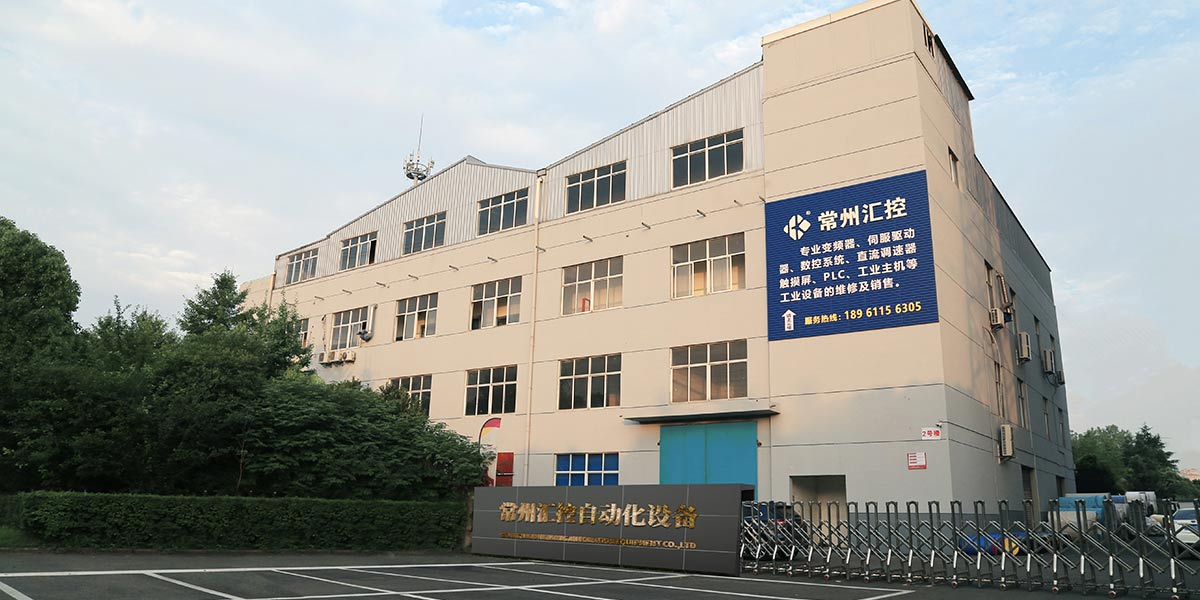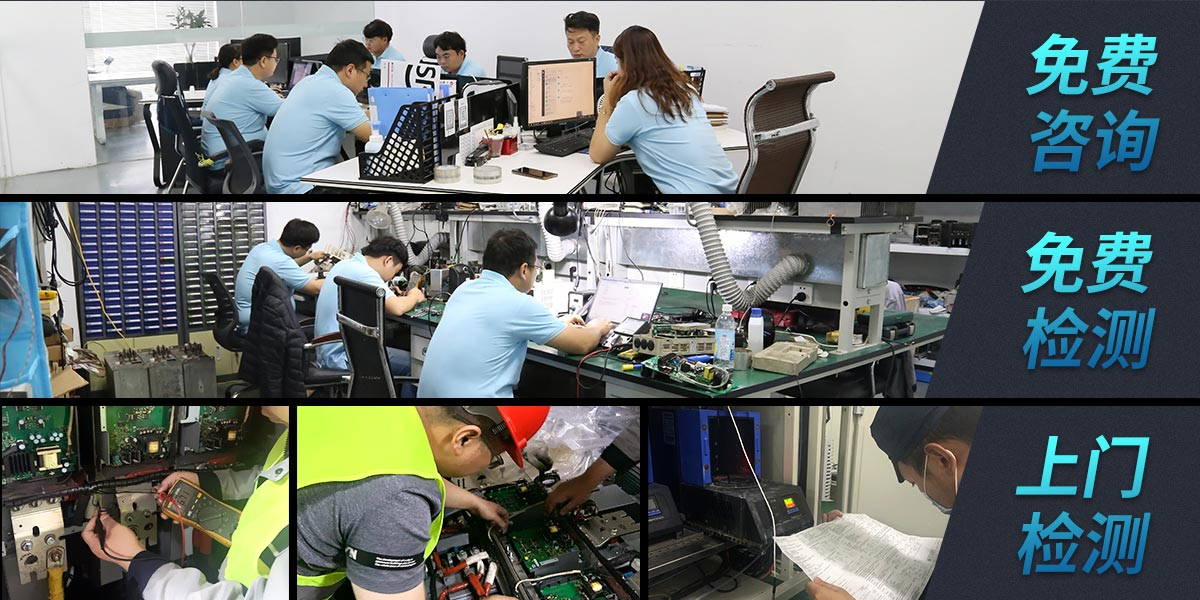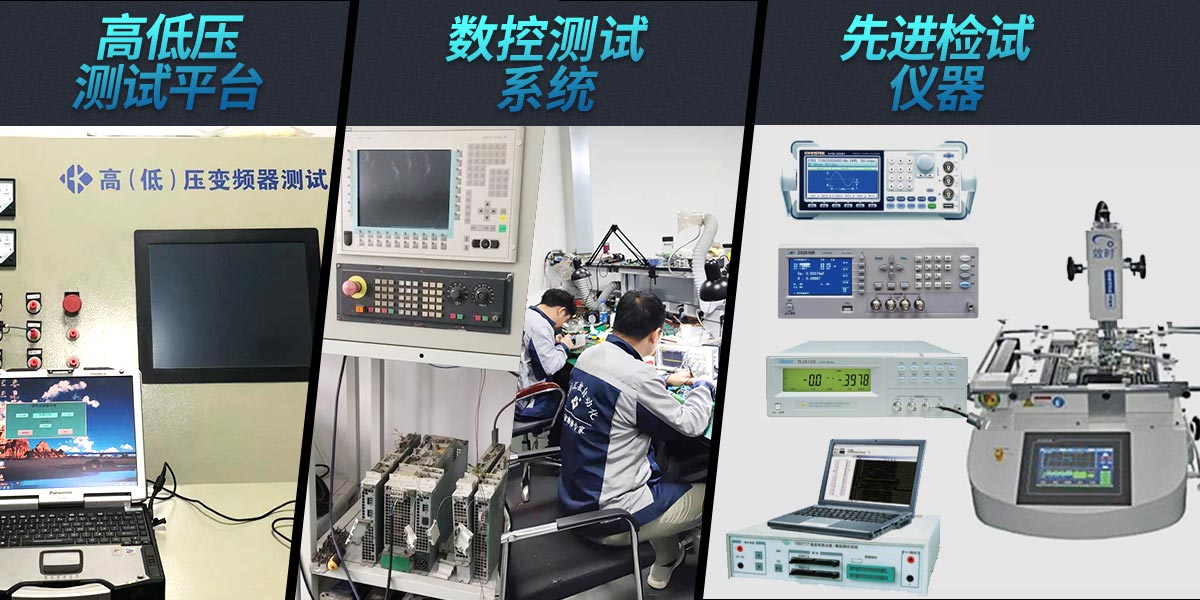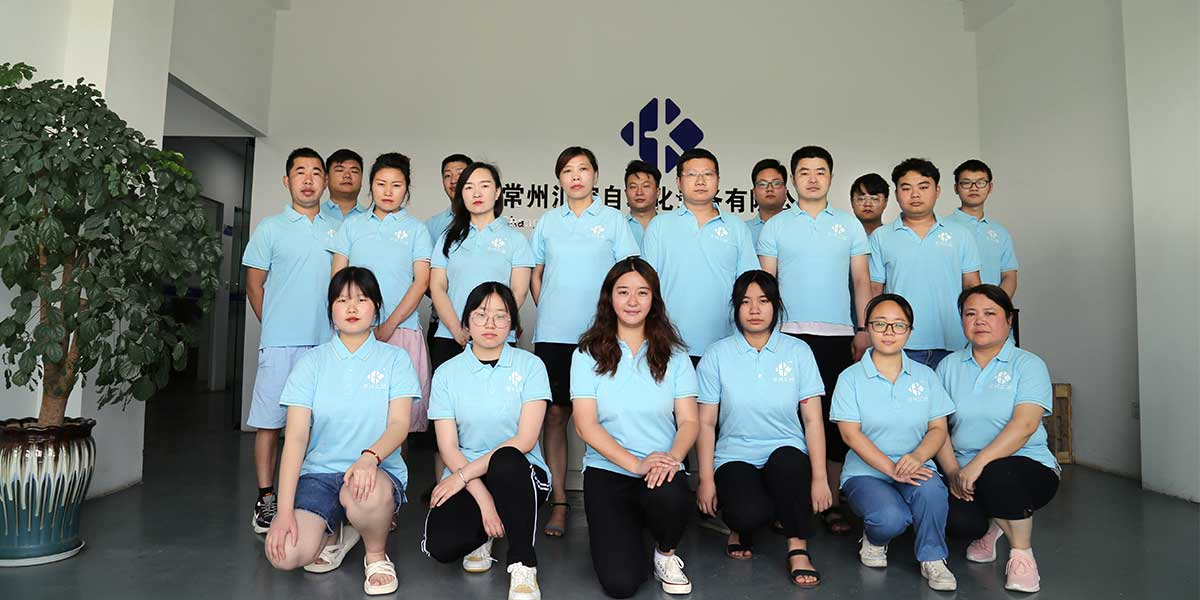# 安川变频器L1000A调试资料

WVF5同步系统配安川L1000A变频器参数设置表

1．然后用“初始化”参数A1-03=2220，将变频器参数初始化。

2．按下表设置变频器参数，设置o1-03=2，o1-04=1。

WVF5同步系统配安川L1000A变频器参数设置表

WVF5同步系统配安川L1000A变频器参数设置表

PLC+L7（带*为G7参数），E参数仅供参考

B3H+L7（带*为G7参数），E参数仅供参考

### 工控维修中心，认准常州汇控20多年维修经验，60多名专业工程师！• 西门子
• ABB
• 安川
• 松下
• 富士
• 施耐德
• 艾默生CT
• SEW
• 三菱
• 丹佛斯
• 台达
• 台安
• 东元
• LS(LG)
• 汇川
• 英威腾
• 海利普
• 普传
• 康沃
• 安邦信
• 易能
• 伟肯
• 东芝
• 士林
• 科比
• 伦茨Lenze
• 罗克韦尔
• 日立
• 森兰
• 伟创
• 四方
• 博世力士乐
• 正弦
• 阿尔法
• 欧姆龙
• 三垦
• 欧陆
• 派克Parker
• 发那科FANUC
• 海德汉
• 贝加莱
• 科尔摩根
• LUST
• 埃斯顿
• 海泰克
• proface
• 宝元
• 新代
• 发格FAGOR
• NUM
• 哈斯HAAS
• 大隈OKUMA
• 报价

• 南京
• 无锡
• 徐州
• 常州
• 苏州
• 青岛
• 南通
• 连云港
• 淮安
• 盐城
• 扬州
• 镇江
• 泰州
• 宿迁
• 江阴
• 宜兴
• 张家港
• 常熟
• 丹阳
• 溧阳
• 句容
• 溧水
• 高淳
• 金坛
• 靖江
• 咨询

x